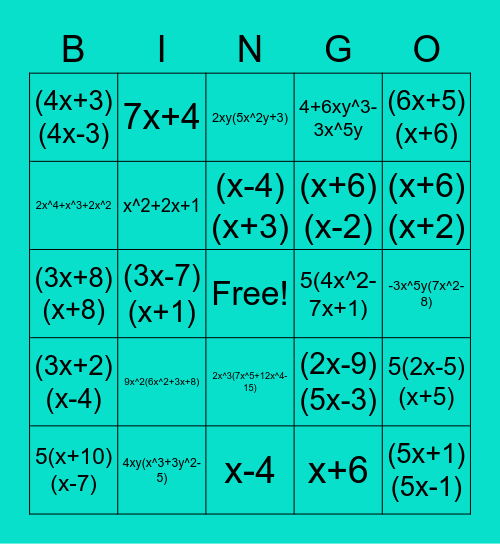# FactoringThis bingo card has a free space and 24 words: 9x^2(6x^2+3x+8), 4xy(x^3+3y^2-5), (x-4)(x+3), 5(x+10)(x-7), (6x+5)(x+6), (4x+3)(4x-3), 2x^4+x^3+2x^2, 7x+4, 5(4x^2-7x+1), 2x^3(7x^5+12x^4-15), (x+6)(x-2), (3x+8)(x+8), (2x-9)(5x-3), 5(2x-5)(x+5), x^2+2x+1, x+6, -3x^5y(7x^2-8), 2xy(5x^2y+3), (x+6)(x+2), (3x-7)(x+1), (5x+1)(5x-1), (3x+2)(x-4), 4+6xy^3-3x^5y and x-4.

## Play Online